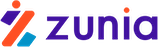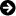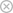Tuyển sinh 2023 dành cho Gen-Z# Graphs. the degree of a vertex

Xem 1-6 trên 6 kết quả Graphs. the degree of a vertex
• ### Lecture Design and Analysis of Algorithms: Lecture 27 - Dr. Sohail Aslam

The main contents of "Lecture Design and Analysis of Algorithms: Lecture 27" include all of the following: Fractional knapsack problem, introduction to graphs, graphs and digraphs, incident edge, degree of a vertex, adjacent vertices, fractional knapsack problem.31pyouzhangjing_190928-04-202281Download

• ### Lecture Design and Analysis of Algorithms: Lecture 28 - Dr. Sohail Aslam

The main contents of "Lecture Design and Analysis of Algorithms: Lecture 28" include all of the following: Degree of a vertex, observations about graphs, paths and cycles, graph representations, shortest path.18pyouzhangjing_190928-04-202281Download

• ### A computationally lightweight and localized centrality metric in lieu of betweenness centrality for complex network analysis

The betweenness centrality (BWC) of a vertex is a measure of the fraction of shortest paths between any two vertices going through the vertex and is one of the widely used shortest path-based centrality metrics for the complex network analysis.16pvititan271113-08-201971Download

• ### Reinhard Diestel Graph Theory

This is an electronic version of the second (2000) edition of the above Springer book, from their series Graduate Texts in Mathematics, vol. 173. The cross-references in the text and in the margins are active links: click on them to be taken to the appropriate page. The printed edition of this book can be ordered from your bookseller, or electronically from Springer through the Web sites referred to below.322pkinhdo090811-10-20126513Download

• ### Báo cáo khoa học:Propagation of mean degrees Dieter Rautenbach

We propose two alternative measures of the local irregularity of a graph in terms of its vertex degrees and relate these measures to the order and the global irregularity of the graph measured by the difference of its maximum and minimum vertex degree.All graphs will be simple and finite. Let G = (V,E) be a graph of order n = |V |. The degree and the neighbourhood of a vertex u 2 V will be denoted by d(u) and N(u). The maximum and minimum degree of G will be denoted by (G) and (G).6pthulanh512-09-2011492Download

• ### Mechanism Design - Enumeration of Kinematic Structures According to Function P6

Classiﬁcation of Mechanisms Using graph representation, mechanism structures can be conveniently represented by graphs. The classiﬁcation problem can be transformed into an enumeration of nonisomorphic graphs for a prescribed number of degrees of freedom, number of loops, number of vertices, and number of edges. The degrees of freedom of a mechanism are governed by Equation (4.3). The number of loops, number of links, and number of joints in a mechanism are related by Euler’s equation, Equation (4.5). The loop mobility criterion is given by Equation (4.7).30pgoixanh27-09-2010775Download

## CHỦ ĐỀ BẠN MUỐN TÌM